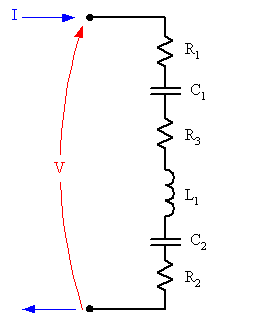# Combining Resistors and Capacitors

megaspazz

## Homework Statement

This is like related to a homework problem, but I'm just curious: If we have two capacitors and two resistors in series, the overall capacitance and resistance does not change regardless of the order, right? Likewise, if they were in parallel (like a resistor + capacitor in series in both branches of the parallel circuit), if you switched the two resistors or the two capacitors, will it cause the overall capacitance and resistance to change?

If you guys don't understand the question, I can draw and upload.

EDIT: I guess another thing I was wondering was: if I had a series circuit that was RCRCRCRC..., could I combine all of the R's in series and C's in series to make a single >---Rnet---Cnet---> circuit? If so, that would also mean that it's the same as the >---Cnet---Rnet---> circuit, right?

## Homework Equations

R = R1 + R2 + R3 + ... (series R)
1/R = 1/R1 + 1/R2 + ... (parallel R)
C = C1 + C2 + C3 + ... (parallel C)
1/C = 1/C1 + 1/C2 + 1/C3 + ... (series C)
-- but I'm not too interested in quantitative!

## The Attempt at a Solution

I know if the series circuit was in RRCC, swapping the two resistors is OK since they're already in series with each other. However, if it were in RCRC, would that be the same as the RRCC or CRRC, etc.?

Staff Emeritus
Homework Helper
Gold Member

## Homework Statement

This is like related to a homework problem, but I'm just curious: If we have two capacitors and two resistors in series, the overall capacitance and resistance does not change regardless of the order, right? Likewise, if they were in parallel (like a resistor + capacitor in series in both branches of the parallel circuit), if you switched the two resistors or the two capacitors, will it cause the overall capacitance and resistance to change?

If you guys don't understand the question, I can draw and upload.

EDIT: I guess another thing I was wondering was: if I had a series circuit that was RCRCRCRC..., could I combine all of the R's in series and C's in series to make a single >---Rnet---Cnet---> circuit? If so, that would also mean that it's the same as the >---Cnet---Rnet---> circuit, right?

## Homework Equations

R = R1 + R2 + R3 + ... (series R)
1/R = 1/R1 + 1/R2 + ... (parallel R)
C = C1 + C2 + C3 + ... (parallel C)
1/C = 1/C1 + 1/C2 + 1/C3 + ... (series C)
-- but I'm not too interested in quantitative!

## The Attempt at a Solution

I know if the series circuit was in RRCC, swapping the two resistors is OK since they're already in series with each other. However, if it were in RCRC, would that be the same as the RRCC or CRRC, etc.?

Do you know Kirchhoff's Laws?

megaspazz
Doesn't that just say the current at each junction is constant, and the voltage in each loop is zero? I am not too sure where you are going with this, though...

megaspazz
Bump?

Mentor
Suppose you have a circuit branch consisting of some combination of R, L, C, components in series. Now suppose that some current I is being injected through that branch:Can you write an expression for the sum of potential drops across the branch? That is, given current I, what's the voltage V across the branch with respect to time? (Assume no initial currents or charges at time t=0).

Now, if you were to use the same components but reorder them and then you were to find the same sum again, would there be any algebraic difference between the first expression and this new one?